Next: Dependency Preservation Up: Desirable Properties of Decomposition Previous: Desirable Properties of Decomposition

Lossless-Join Decomposition

1. We claim the above decomposition is lossless. How can we decide whether a decomposition is lossless?

• Let R be a relation schema.
• Let F be a set of functional dependencies on R.
• Let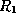and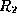form a decomposition of R.
• The decomposition is a lossless-join decomposition of R if at least one of the following functional dependencies are in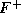:
1.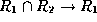2.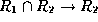Why is this true? Simply put, it ensures that the attributes involved in the natural join (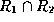) are a candidate key for at least one of the two relations.

This ensures that we can never get the situation where spurious tuples are generated, as for any value on the join attributes there will be a unique tuple in one of the relations.

2. We'll now show our decomposition is lossless-join by showing a set of steps that generate the decomposition:
• First we decompose Lending-schema into

Branch-schema = (bname, bcity, assets)

Loan-info-schema = (bname, cname, loan#, amount)

• Since bnameassets bcity, the augmentation rule for functional dependencies implies that

bnamebname assets bcity

• Since Branch-schema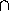Borrow-schema = bname, our decomposition is lossless join.
• Next we decompose Borrow-schema into

Loan-schema = (bname, loan#, amount)

Borrow-schema = (cname, loan#)

• As loan# is the common attribute, and

loan#amount bname

This is also a lossless-join decomposition.

Osmar Zaiane
Thu Jun 18 12:56:34 PDT 1998